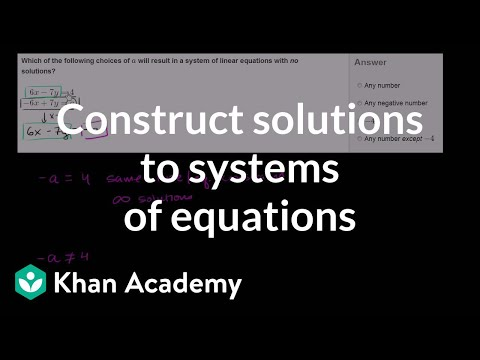Video

# Systems of equations with graphing: 5x + 3y = 7 and 3x - 2y = 8 (Full video)

Description: Sal demonstrates how to solve the system of equations 5x + 3y = 7 and 3x - 2y = 8 by graphing. 5x plus 3y is equal to 7, and 3x minus 2y is equal to 8. So if we were looking for an x and a y that satisfies both, that point needs to be on both equations or it has to be on both graphs. So let's focus on this first equation, and I want to graph it.

### Other videos you might be interested in### Completing solutions to 2-variable equations (Full video)